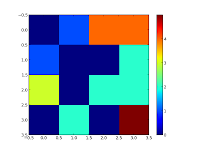## Friday, June 29, 2012

### Some code available on github

I started to push some code on github

## Tuesday, June 26, 2012

### Getting the neighborhood of labelled particles in a graph

In the previous post, a labelled image, typically obtained by labelling connected components of a binary image, was used to compute a dictionary representing the neighborhood relationships between the particles.
A dictionary may be not the best data structure to represent such relationship. A graph structure is better suited and networkx provides a python implementation for that.
Starting from the following label image:Background Grey level=0, first particle: grey level=1, ...last particle:grey level=5

We get two possible graphs. The first one takes the background (vertex 0) into account so the background is a neighbour of all the particles (there an edge between the vertex 0 and all the other vertex)

In this second graph, the background was removed:

A function  converts the dictionary to a networkx graph:

```# -*- coding: utf-8 -*-
"""
Created on Mon Jun 25 10:26:01 2012

@author: Jean-Patrick Pommier
"""

import numpy as np
import networkx as nx
import mahotas as mh
import pylab as plb

def makelabelarray():
label = np.array([[0,1,4,4],
[1,0,0,2],
[3,0,2,2],
[0,2,0,5]])
return label

def convertToGraph(dic, noBack=True):
G = nx.Graph()
G.add_nodes_from(dic.keys())
for particle in dic.keys():
list_touching_particles = dic[particle]
# remove background
if noBack:
list_touching_particles.discard(0)
print 'v(',particle,')=',list_touching_particles
for tp in list_touching_particles:
G.add_edge(particle,tp)
return G

def findneighborhoods(label,neighborhood):
''' given a labelled image, should return the adjacency list
of particles, for a given neighborhood:

neighborhood=np.array([0,1,0],[1,1,1],[0,1,0])

The background (0), is kept as a particle neighbor
No fancy indexing
'''
#make the labels list
labmax = label.max()
#print labmax
neighb_dic = {} # a dictionnary containing particle label as key and neighborhood
for i in range(1,labmax+1):
mask = (label ==i)
#print mask
dilated = mh.dilate(mask,neighborhood)
neighbor = np.logical_and(dilated, np.logical_not(mask))
#print neighbor
#=======================================================================
flatlab = np.ndarray.flatten(label)
flatneighborhood = np.ndarray.flatten(neighbor)
flatneighbors = flatlab[flatneighborhood]
flatneighbors.sort()
#set is a trick so that each value of the neighborhoods is present only once
neighb_dic[i] = set(flatneighbors)
#print np.nonzero(flatneighbors)
return neighb_dic

if __name__ == "__main__":
a = makelabelarray()
n = np.array([[1,1,1],[1,1,1],[1,1,1]])
g = findneighborhoods(a, n)
G = convertToGraph(g, noBack=True)

plb.imshow(a,interpolation = 'nearest')
plb.colorbar(ticks=[0,1,2,3,4])
plb.show()

nx.draw(G)
plb.show()

```

## Monday, June 25, 2012

### Neighbourhood in a labelled image

Image segmentation can yield labelled images, where the different labels correspond to the objects of interest ( chromosomes, a nuclei ).

It can be necessary to consider particles in the neighbourhood of a given particle.
The following 4x4 labelled image (a numpy array) is made of four particles:
• first particle : light blue (value or label=1)
• second particle : green (value=2)
• third particle : orange (value=3)
• fourth particle : red (value=4)
• background : dark blue (value = 0)

Considering a 3x3 domain (in fact a structuring element), the neighbour particle of the first particle is the 'orange' particle. The 'orange' particle has two neighbours, the 'light blue' and the 'green' particle .

The following python script takes a label image (from the above example) and returns a dictionary, where  in the  key/value pair, the key corresponds to a chosen particle and the value to a set containing the label value of the neighbouring particles:

{1: set([0, 3]), 2: set([0, 3, 4]), 3: set([0, 1, 2]), 4: set([0, 2])}
The calculated dictionary is closed to an adjacency list.

```# -*- coding: utf-8 -*-
"""
Created on Mon Jun 25 10:26:01 2012

@author: Jean-Patrick Pommier
"""

import numpy as np
import mahotas as mh
import pylab as plb

def makelabelarray():
label = np.array([[0,1,0,0],
[1,0,0,2],
[3,0,2,2],
[0,2,0,4]])
return label

def findneighborhoods(label,neighborhood):
''' given a labelled image, should return the adjacency list
of particles, for a given neighborhood:

neighborhood=np.array([1,1,1],[1,1,1],[1,1,1])

The background (0), is kept as a particle neighbor
No fancy indexing
'''
#make the labels list
labmax = label.max()
#print labmax
neighb_dic = {} # a dictionnary containing particle label as key and neighborhood
for i in range(1,labmax+1):
mask = (label ==i)
#print mask
dilated = mh.dilate(mask,n)
neighbor = np.logical_and(dilated, np.logical_not(mask))
#print neighbor
#==============================================================================
#         #Done without fancy indexing
#==============================================================================
flatlab = np.ndarray.flatten(label)
flatneighborhood = np.ndarray.flatten(neighbor)
flatneighbors = flatlab[flatneighborhood]
flatneighbors.sort()
#set is a trick so that each value of the neighborhoods is present only once
neighb_dic[i] = set(flatneighbors)
#print np.nonzero(flatneighbors)
return neighb_dic

if __name__ == "__main__":
a = makelabelarray()
n = np.array([[1,1,1],[1,1,1],[1,1,1]])
print findneighborhoods(a, n)
plb.imshow(a,interpolation = 'nearest')
plb.show()

```

## Saturday, June 23, 2012

### cv2 opens a 12 bits TIF image properly

I tried to open a 12 bits TIFF image with python opencv (cv2) as follow:

```# -*- coding: utf-8 -*-
"""
Created on Wed Jun 20 09:35:31 2012

"""
import cv2
import skimage.io as sio
png = cv2.imread('/home/simon/QFISH/JPPAnimal/JPP52/11/DAPI/particles/part9.png')
tif = cv2.imread('/home/simon/QFISH/JPPAnimal/JPP52/11/DAPI/1.TIF')
tiff = sio.imread('/home/simon/QFISH/JPPAnimal/JPP52/11/DAPI/1.TIF')
print png.dtype, type(png)
print tif.dtype, type(tif)
print tiff.dtype, type(tiff)
sio.imshow(tiff)
sio.show()
```

cv2 recognises the image 1.TIF (12 bits TIFF) as 8 bits image, as the console output is:

uint8 <type 'numpy.ndarray'>
uint8 <type 'numpy.ndarray'>
uint16 <type 'numpy.ndarray'>

may be a flag issue in cv2.imread...

From Stackoverflow, or from the cv2 doc,  the image must be loaded "as it is" with a flag set to -1:
`tif = cv2.imread('/home/simon/QFISH/JPPAnimal/JPP52/11/DAPI/1.TIF', -1)`

The image loaded with cv2.imread can be then displayed as any numpy.ndarray with skimage: